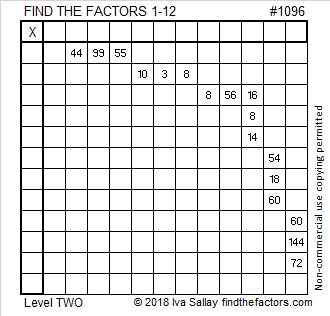# 1096 and Level 2

There are 17 clues in this level 2 puzzle. Two of those clues are 60 and three of them are 8. In a regular 12 × 12 multiplication table, both of those numbers appear 4 times each. The factors for this multiplication table puzzle won’t be in the usual places. Can you figure out where they need to go?Print the puzzles or type the solution in this excel file: 12 factors 1095-1101

Here is a little bit about the number 1096:

• 1096 is a composite number.
• Prime factorization: 1096 = 2 × 2 × 2 × 137, which can be written 1096 = 2³ × 137
• The exponents in the prime factorization are 1 and 3. Adding one to each and multiplying we get (3 + 1)(1 + 1) = 4 × 2 = 8. Therefore 1096 has exactly 8 factors.
• Factors of 1096: 1, 2, 4, 8, 137, 274, 548, 1096
• Factor pairs: 1096 = 1 × 1096, 2 × 548, 4 × 274, or 8 × 137
• Taking the factor pair with the largest square number factor, we get √1096 = (√4)(√274) = 2√274 ≈ 33.105891096 is the hypotenuse of one Pythagorean triple:
704-840-1096 which is 8 times (88-105-137)